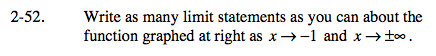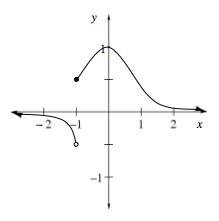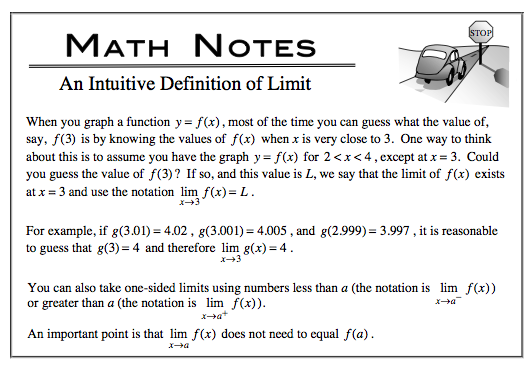### Home > CALC > Chapter Ch2 > Lesson 2.2.1 > Problem2-52

2-52.Limits give information about the location of holes and asymptotes, because they are predicted values, not real values.$\lim_{x\rightarrow 1^{-}}f(x)=$

$\lim_{x\rightarrow 1^{+}}f(x)=$

$\lim_{x\rightarrow -\infty }f(x)=$

$\lim_{x\rightarrow \infty }f(x)=$